# Medial Axis Calculation

### Introduction:

Medial Axis is an important tool for many applications including shape detection, domain decomposition,  and geometric compression. In this homework assignment, I have created 2D medial axis calculation using Jonathan Shewchuck's Constrained Delaunay Triangulation ( Triangle) and robust geometric predicates ( including robust circumcenter calculation).

A Pseudo code for Medial Axis is as follows

void MedialAxis( boundaryNodes, medialNodes, medialEdges)
{
// Input:
//  vector<Point2D>  boundaryNodes;  in the anticlockwise direction. In this example we assume that the polygon is closed
//                                                                   and simple connected;
//  Output:
//  vector<Point2D>  medialNodes;          The coordinates of the medial points generated.
//  map<int,int>        medialEdges;          The edges forming the medial axis;
//
//  Step: I; using Jonathan Shewchuk's triangle with -pg option. i.e.

vector<Triangle> cdt = getConstrainedTriangulation ( boundaryNodes );

//  Step 2:  Calculate the circumcenter using Jonathan Shewchuk's exact Circumcenter software

nTriangles  = cdt.size();
for ( size_t i = 0; i < numTriangles; i++)
{
Triangle src = cdt[i];
Point2D  c = getCircumCenter( src );
// Check that point 'C' is within some triangle of the CDT i.e. it does not fall outside
bool found  = getHoldingTriangle( cdr, c, dst );
if( found ){
medialPoint.src  = src;
medialPoint.dst  = dst;
medialPoint.center =  c;
}
}

//Step 3:  Get all the edges of CDT;
vector<Edge> meshedges = getEdges( cdt);
size_t numEdges = meshedges.size();
for( size_t i = 0; i < numEdges; i++)
vector<Triangle>  edgefaces = getFaceNeighbours( meshedge[i] );
if( edgeface.size() == 2) {
bool found0 = getMedialPoint( edgeface,  mp0 );
bool found1 =  getMedialPoint( edgeface, mp1 );
if( found0 && found1 )
medialEdges.push_back( make_pair(mp0.id, mp1,id);
}
}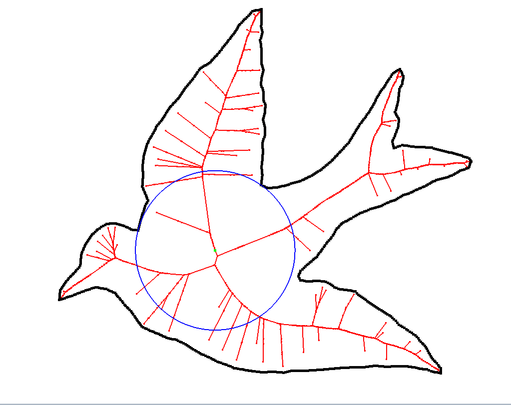Download DataSet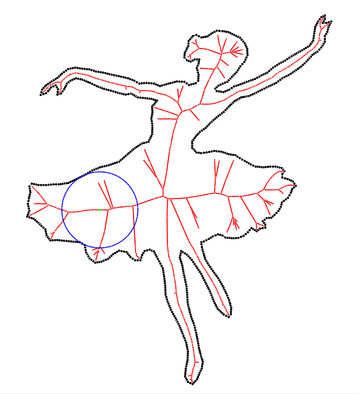Download Dataset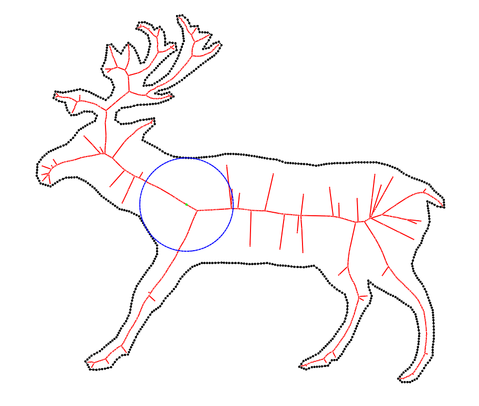Download Dataset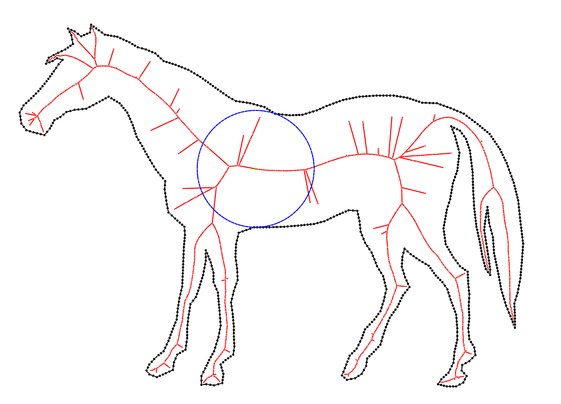Download dataset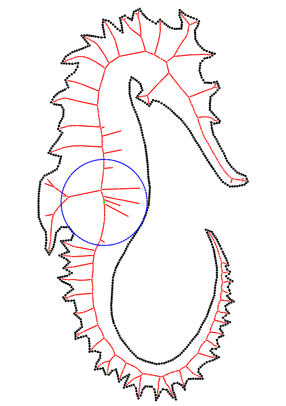Download dataset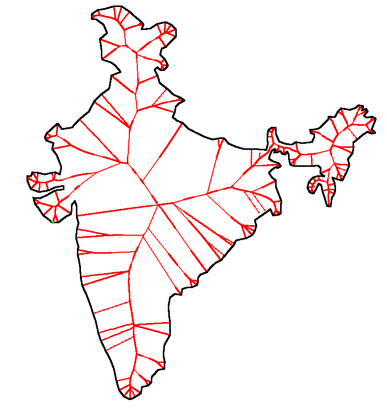Download dataset